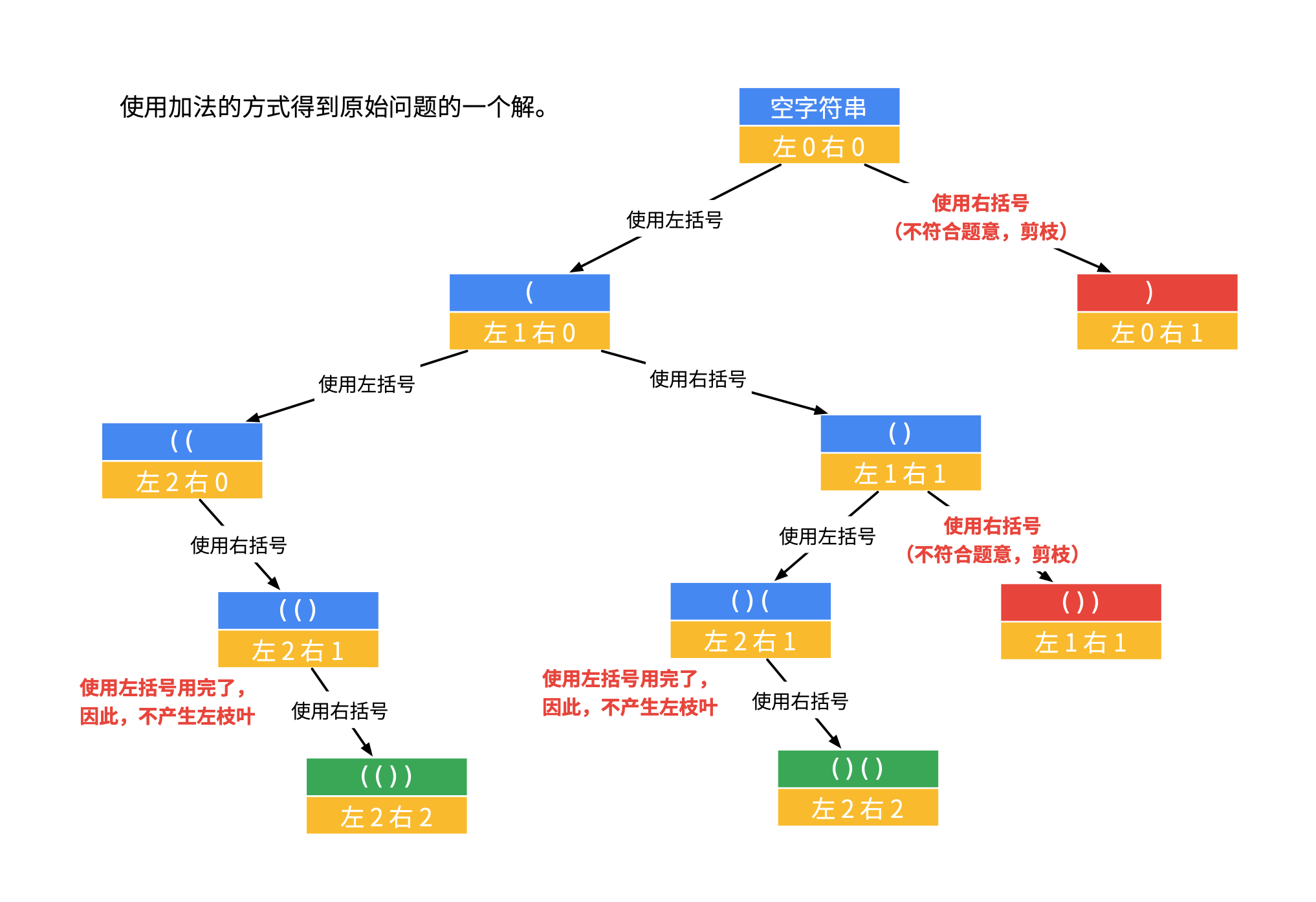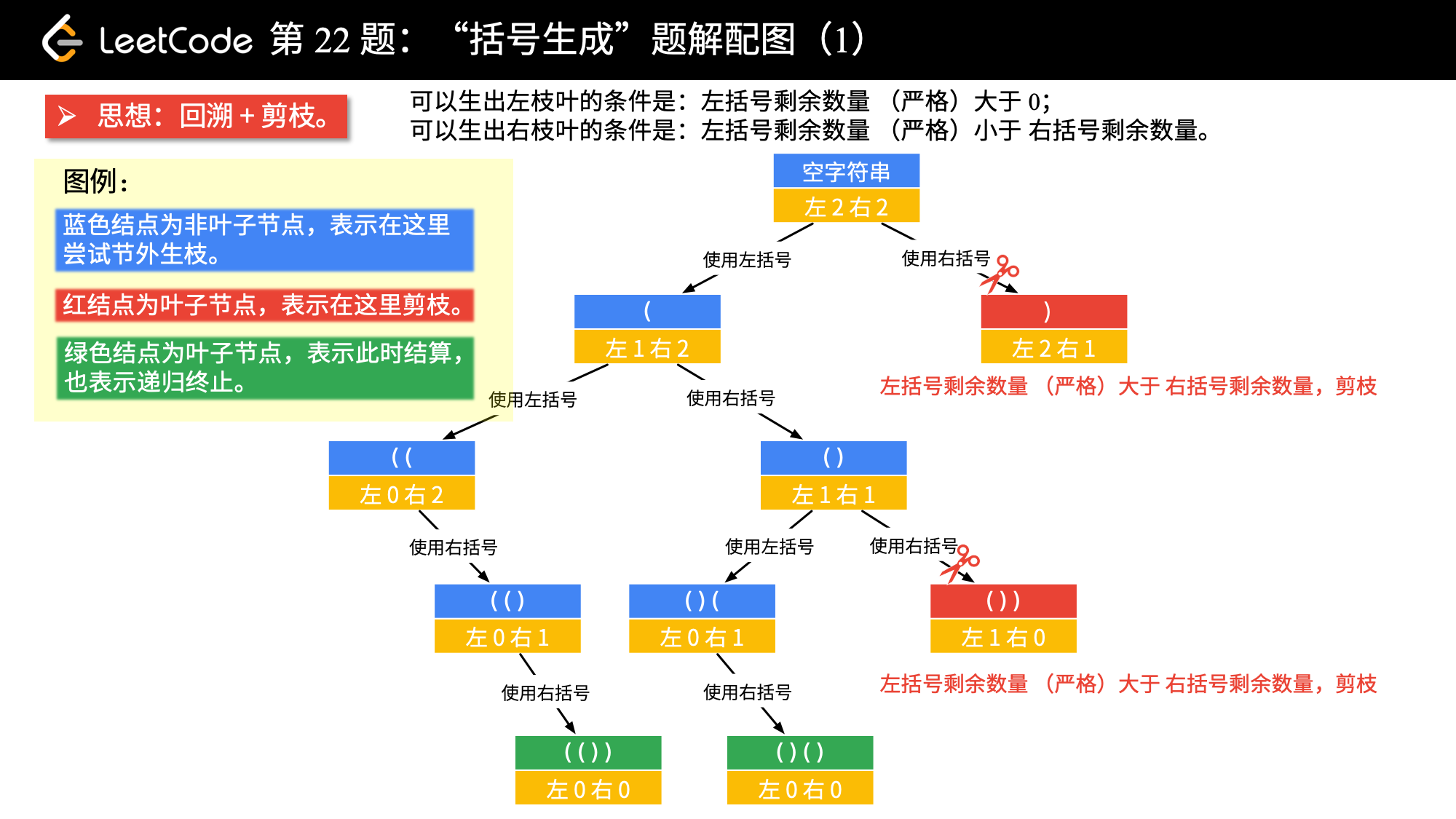# 20. 有效的括号

## 题目

1. 左括号必须用相同类型的右括号闭合。

2. 左括号必须以正确的顺序闭合。

3. 每个右括号都有一个对应的相同类型的左括号。

### 例子

输入：s = "()"



输入：s = "()[]{}"



输入：s = "(]"



### 提示：

1 <= s.length <= 10^4

s 仅由括号 '()[]{}' 组成

## V1-基于 Stack

### 思路

### java 实现

java
import java.util.HashMap;
import java.util.Map;
import java.util.Stack;

/**
* @author d
* @since 1.0.0
*/
public class T020_ValidParentheses {

private static final Map<Character, Character> MAP = new HashMap<>(4);

static {
MAP.put(')', '(');
MAP.put('}', '{');
MAP.put(']', '[');
}

/**
* 简单思路
* @param s 字符串
* @return 结果
* @since v1
*/
public boolean isValid(String s) {
if(null == s || s.length() == 0) {
return true;
}
// 奇数
if(s.length() % 2 != 0) {
return false;
}

Stack<Character> stack = new Stack<>();
for(int i = 0; i < s.length(); i++) {
char c = s.charAt(i);

if(c == '(' || c == '{' || c == '[') {
stack.push(c);
} else {
// 开始 pop
if(stack.isEmpty()) {
return false;
}

char pop = stack.pop();
char expectPop = MAP.get(c);

if(pop != expectPop) {
return false;
}
}

}

return stack.isEmpty();
}

}


### 效果

Runtime: 1 ms, faster than 98.79% of Java online submissions for Valid Parentheses.
Memory Usage: 37.4 MB, less than 70.07% of Java online submissions for Valid Parentheses.


## v2-模拟 stack

### java 实现

    /**
* 大道至简
*
* @param s 字符串
* @return 结果
* @since v1
*/
public boolean isValid(String s) {
int length = s.length();
char[] stack = new char[length];

for(int i = 0; i < length; i++) {
char c = s.charAt(i);

switch (c) {
case '{':
case '[':
case '(':
break;
case '}':
return false;
}
break;
case ']':
return false;
}
break;
case ')':
return false;
}
break;
}
}

}


### 效果

Runtime: 0 ms, faster than 100.00% of Java online submissions for Valid Parentheses.
Memory Usage: 37.1 MB, less than 95.67% of Java online submissions for Valid Parentheses.


# 22. 括号生成

## 题目

输入：n = 3

"((()))",
"(()())",
"(())()",
"()(())",
"()()()"
]


## 思路1-暴力破万法

### 流程

1. 构建所有 () 可能构成的字符串

2. 过滤出其中符合条件的字符串。

### 示例代码

/**
*
* 思路：
*
* （1）生成所有可能
* （2）如果符合则加入结果
*
* @param n 数字
* @return 结果
*/
public List<String> generateParenthesis(int n) {
List<String> all = generateAll(n);
List<String> resultList = new ArrayList<>();
for(String string : all) {
if(isValid(string)) {
}
}
return resultList;
}

/**
* 生成所有的可能字符串
* @param n 个数
* @return 结果
*/
private List<String> generateAll(final int n) {
List<String> resultList = new ArrayList<>();
char[] chars = new char[]{'(', ')'};
for(int i = 0; i < n*2; i++) {
List<String> newList = new ArrayList<>();
for(String result : resultList) {
for(char c: chars) {
}
}
resultList = newList;
}
return resultList;
}

/**
* 是否合法
* @param s 字符串
* @return 结果
*/
private boolean isValid(final String s) {
int length = s.length();
for(int i = 0; i < length; i++) {
char c = s.charAt(i);
if('(' == c) {
} else {
return false;
}
}
}
}


## 思路2-DFS 深度优先遍历### 流程

1. 第一个有效符号一定是 (

2. ( 个数比 ) 少的时候，进行剪枝

3. () 的个数都等于 n 的时候，一次遍历就结束了。

### 实现

public List<String> generateParenthesis(int n) {
List<String> resultList = new ArrayList<>();
dfs(resultList, "", 0, 0, n);
return resultList;
}

/**
* 深度优先遍历
* @param resultList 结果列表
* @param string 字符串
* @param left 左括号
* @param right 右括号
* @param num 位数
*/
private void dfs(List<String> resultList,
String string,
int left,
int right,
int num) {
if(left == num && right == num) {
return;
}
//左边的括号是可以一直加的
if(left < num) {
dfs(resultList, string+"(", left+1, right, num);
}
// 这里是对剪枝处理的优化，如果 left < right，是跳过的。
// ) 小于 < 的时候，可以添加
if(right < left) {
dfs(resultList, string+")", left, right+1, num);
}
}


### 性能

Runtime: 1 ms, faster than 85.42% of Java online submissions for Generate Parentheses.
Memory Usage: 39 MB, less than 98.86% of Java online submissions for Generate Parentheses.


## 思路3-DFS 深度优先遍历改进

### 实现

public List<String> generateParenthesis(int n) {
List<String> resultList = new ArrayList<>();
StringBuilder stringBuilder = new StringBuilder(n << 1);
backtrack(resultList, stringBuilder, 0, 0, n);
return resultList;
}

/**
* 递归处理
* @param resultList 结果列表
* @param stringBuilder 字符串
* @param left 左括号
* @param right 右括号
* @param num 位数
*/
private void backtrack(List<String> resultList,
StringBuilder stringBuilder,
int left,
int right,
int num) {
if(stringBuilder.length() == num << 1) {
return;
}
//左边的括号是可以一直加的
if(left < num) {
backtrack(resultList, stringBuilder.append("("), left+1, right, num);
// 重新设置
stringBuilder.setLength(stringBuilder.length()-1);
}
// ) 小于 < 的时候，可以添加
if(right < left) {
backtrack(resultList, stringBuilder.append(")"), left, right+1, num);
stringBuilder.setLength(stringBuilder.length()-1);
}
}


### 性能

Runtime: 0 ms, faster than 100.00% of Java online submissions for Generate Parentheses.
Memory Usage: 39.6 MB, less than 63.29% of Java online submissions for Generate Parentheses.


## 思路4-DFS 深度优先方式2### java 实现

public List<String> generateParenthesis(int n) {
List<String> resultList = new ArrayList<>();
dfs(resultList, "", n, n, n);
return resultList;
}

/**
* 深度优先遍历
* @param resultList 结果列表
* @param string 字符串
* @param left 左括号
* @param right 右括号
* @param num 位数
*/
private void dfs(List<String> resultList,
String string,
int left,
int right,
int num) {
if(left == 0 && right == 0) {
return;
}
// 剪枝（左括号可以使用的个数严格大于右括号可以使用的个数）
if (left > right) {
return;
}
if(left > 0) {
dfs(resultList, string+"(", left-1, right, num);
}
if(right > 0) {
dfs(resultList, string+")", left, right-1, num);
}
}


## 思路5-BFS 广度优先遍历

### java 实现

class Node {
private String text;
private int left;
private int right;

public Node(String text, int left, int right) {
this.text = text;
this.left = left;
this.right = right;
}
}

/**
* @param n 数字
* @return 结果
*/
public List<String> generateParenthesis(int n) {
List<String> res = new ArrayList<>();
if (n == 0) {
return res;
}
// 从上到下
Node rootNode = new Node("", 0, 0);
while (!queue.isEmpty()) {
Node curNode = queue.remove();
// 最后一层
if (curNode.left == n && curNode.right == n) {
}
if (curNode.left < n) {
queue.add(new Node(curNode.text + "(", curNode.left + 1, curNode.right));
}
// 剪枝
if (curNode.right < curNode.left) {
queue.add(new Node(curNode.text + ")", curNode.left, curNode.right + 1));
}
}
return res;
}


## 思路6-DP 动态规划

### 核心思路

1. 我们需要枚举与第一个 ( 对应的 ) 的位置 2 * i + 1；

2. 递归调用 generate(i) 即可计算 a 的所有可能性；

3. 递归调用 generate(n - i - 1) 即可计算 b 的所有可能性；

4. 遍历 a 与 b 的所有可能性并拼接，即可得到所有长度为 2 * n 的括号序列。

ps: DP 最核心的两点

（1）找到递推公式

（2）缓存结果，避免重复计算

### java 实现

public List<String> generateParenthesis(int n) {
// 存放缓存信息
List<List<String>> cache = new ArrayList<>();
// 初始化第一个元素列表为 [""]
for (int i = 1; i <= n; i++) {
List<String> cur = new ArrayList<>();
for (int j = 0; j < i; j++) {
List<String> str1 = cache.get(j);
List<String> str2 = cache.get(i - 1 - j);
for (String s1 : str1) {
for (String s2 : str2) {
// 枚举右括号的位置
cur.add("(" + s1 + ")" + s2);
}
}
}
}
return cache.get(n);
}


### 性能

Runtime: 7 ms, faster than 13.20% of Java online submissions for Generate Parentheses.
Memory Usage: 39.5 MB, less than 65.23% of Java online submissions for Generate Parentheses.


# 32. 最长有效括号 Longest Valid Parentheses

## 题目

### 例子

输入：s = "(()"



输入：s = ")()())"



输入：s = ""



0 <= s.length <= 3 * 10^4

s[i] 为 '(' 或 ')'

## v1-暴力算法

### 思路

### java 实现

java
/**
* 最简单的思路：
*
* 1. 通过双指针，两边移动。截取 substring
* 2. 通过 020 的方法，判断字符串是否为 valid，是返回 j-i
* 3. i j 重合，返回 0
*
* 这种解法：会在 222 CASE 超时
*
* @param s 字符串
* @return 结果
*/
public int longestValidParentheses(String s) {
//0.1 都不是
if(s.length() <= 1) {
return 0;
}

// 这个复杂度是 o(N^3)，肯定没戏
for (int stepLen = s.length(); stepLen >= 2; stepLen--) {
// 逆序，本质是双指针
for(int i = 0; i < s.length()-1; i++) {
// fast-return
if(i + stepLen > s.length()) {
break;
}

String subString = s.substring(i, i+stepLen);

if(isValid(subString)) {
return stepLen;
}
}
}

// 没有
return 0;
}

/**
* 大道至简
*
* T020
*
* @param s 字符串
* @return 结果
* @since v1
*/
public static boolean isValid(String s) {
// 奇数个，不可能满足
if(s.length() % 2 != 0) {
return false;
}

Stack<Character> stack = new Stack<>();
for(int i = 0; i < s.length(); i++) {
char c = s.charAt(i);

if(c == '(') {
stack.push(c);
} else {
// 开始 pop
if(stack.isEmpty()) {
return false;
}

char pop = stack.pop();
char expectPop = '(';

if(pop != expectPop) {
return false;
}
}

}

return stack.isEmpty();
}


## V2-基于 stack

### 思路

1) 对于遇到的每个 (，我们将它的下标放入栈中

2) 对于遇到的每个 )，我们先弹出栈顶元素表示匹配了当前右括号：

2.1) 如果栈为空，说明当前的右括号为没有被匹配的右括号，我们将其下标放入栈中来更新我们之前提到的「最后一个没有被匹配的右括号的下标」

2.2) 如果栈不为空，当前右括号的下标减去栈顶元素即为「以该右括号为结尾的最长有效括号的长度」

### java 实现

class Solution {
public int longestValidParentheses(String s) {
int maxans = 0;
stack.push(-1);
for (int i = 0; i < s.length(); i++) {
if (s.charAt(i) == '(') {
stack.push(i);
} else {
stack.pop();

if (stack.isEmpty()) {
stack.push(i);
} else {
maxans = Math.max(maxans, i - stack.peek());
}
}
}
return maxans;
}
}


())((()) 为例子，日志为：

i=0, stack=[0, -1], maxans=0
i=1, stack=[-1], maxans=2
i=2, stack=, maxans=2
i=3, stack=[3, 2], maxans=2
i=4, stack=[4, 3, 2], maxans=2
i=5, stack=[5, 4, 3, 2], maxans=2
i=6, stack=[4, 3, 2], maxans=2
i=7, stack=[3, 2], maxans=4


TC：O(N)

MC: O(N)

## v3-DP 动态规划

1）确定状态

2）转移方程

3）初始条件和边界情况

4）计算顺序

### 确定状态-最后一步

1） s[i] == '(' ，这时，s[i] 无法和其之前的元素组成有效的括号对，所以，dp[i] = 0

2) s[i] == ')'，这时，需要看其前面对元素来判断是否有有效括号对。

2.1) 情况1:

s[i - 1] == '('，即 s[i] 和 s[i - 1] 组成一对有效括号，有效括号长度新增长度2，i 位置对最长有效括号长度为 其之前2个位置的最长括号长度加上当前位置新增的2，我们无需知道i-2位置对字符是否可以组成有效括号对。

dp[i] = dp[i - 2] + 2


2.2) 情况2:

s[i - 1] == ')'

dp[i] = dp[i - 1] + 2


dp[i] = dp[i - 1] + dp[i - dp[i - 1] - 2] + 2


### 子问题

dp[i] = dp[i - 2] + 2

dp[i] = dp[i - 1] + dp[i - dp[i - 1] - 2] + 2


### 转移方程

if s[i] == '(' :
dp[i] = 0
if s[i] == ')' :
if s[i - 1] == '(' :
dp[i] = dp[i - 2] + 2 #要保证i - 2 >= 0

if s[i - 1] == ')' and s[i - dp[i - 1] - 1] == '(' :
dp[i] = dp[i - 1] + dp[i - dp[i - 1] - 2] + 2 #要保证i - dp[i - 1] - 2 >= 0


### java 实现

class Solution {
public int longestValidParentheses(String s) {
int maxans = 0;
int[] dp = new int[s.length()];
for (int i = 1; i < s.length(); i++) {
if (s.charAt(i) == ')') {
if (s.charAt(i - 1) == '(') {
dp[i] = (i >= 2 ? dp[i - 2] : 0) + 2;
} else if (i - dp[i - 1] > 0 && s.charAt(i - dp[i - 1] - 1) == '(') {
dp[i] = dp[i - 1] + ((i - dp[i - 1]) >= 2 ? dp[i - dp[i - 1] - 2] : 0) + 2;
}
maxans = Math.max(maxans, dp[i]);
}
}
return maxans;
}
}


ps: 这个递推公式比较难，比较容易出错。

## V4-正向逆向结合

### 思路

1) 当 left 计数器比 right计数器大时，我们将 left 和 right计数器同时变回 0

2) 当 left 计数器与 right计数器相等时，我们计算当前有效字符串的长度，并且记录目前为止找到的最长子字符串

### java 实现

    public int longestValidParentheses(String s) {
int left = 0, right = 0, maxlength = 0;

// 从左往右
for (int i = 0; i < s.length(); i++) {
if (s.charAt(i) == '(') {
left++;
} else {
right++;
}
if (left == right) {
maxlength = Math.max(maxlength, 2 * right);
} else if (right > left) {
left = right = 0;
}
}

// 从右往左
left = right = 0;
for (int i = s.length() - 1; i >= 0; i--) {
if (s.charAt(i) == '(') {
left++;
} else {
right++;
}
if (left == right) {
maxlength = Math.max(maxlength, 2 * left);
} else if (left > right) {
left = right = 0;
}
}
return maxlength;
}


TC: O(N)

MC: O(1)

# 开源地址

https://github.com/houbb/leetcode

# 参考资料

generate-parentheses

https://leetcode.cn/problems/longest-valid-parentheses/solution/zui-chang-you-xiao-gua-hao-by-leetcode-solution/

https://leetcode.cn/problems/longest-valid-parentheses/solution/dong-tai-gui-hua-si-lu-xiang-jie-c-by-zhanganan042/